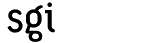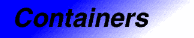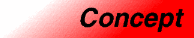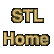# Associative ContainerCategory: containers Component type: concept

### Description

An Associative Container is a variable-sized Container that supports efficient retrieval of elements (values) based on keys. It supports insertion and removal of elements, but differs from a Sequence in that it does not provide a mechanism for inserting an element at a specific position. 

As with all containers, the elements in an Associative Container are of type value_type. Additionally, each element in an Associative Container has a key, of type key_type. In some Associative Containers, Simple Associative Containers, the value_type and key_type are the same: elements are their own keys. In others, the key is some specific part of the value. Since elements are stored according to their keys, it is essential that the key associated with each element is immutable. In Simple Associative Containers this means that the elements themselves are immutable, while in other types of Associative Containers, such as Pair Associative Containers, the elements themselves are mutable but the part of an element that is its key cannot be modified. This means that an Associative Container's value type is not Assignable.

The fact that the value type of an Associative Container is not Assignable has an important consequence: associative containers cannot have mutable iterators. This is simply because a mutable iterator (as defined in the Trivial Iterator requirements) must allow assignment. That is, if i is a mutable iterator and t is an object of i's value type, then *i = t must be a valid expression.

In Simple Associative Containers, where the elements are the keys, the elements are completely immutable; the nested types iterator and const_iterator are therefore the same. Other types of associative containers, however, do have mutable elements, and do provide iterators through which elements can be modified. Pair Associative Containers, for example, have two different nested types iterator and const_iterator. Even in this case, iterator is not a mutable iterator: as explained above, it does not provide the expression *i = t. It is, however, possible to modify an element through such an iterator: if, for example, i is of type map<int, double>, then (*i).second = 3 is a valid expression.

In some associative containers, Unique Associative Containers, it is guaranteed that no two elements have the same key.  In other associative containers, Multiple Associative Containers, multiple elements with the same key are permitted.

### Refinement of

Forward Container, Default Constructible

### Associated types

One new type is introduced, in addition to the types defined in the Forward Container requirements.
 Key type X::key_type The type of the key associated with X::value_type. Note that the key type and value type might be the same.

### Notation

 X A type that is a model of Associative Container a Object of type X t Object of type X::value_type k Object of type X::key_type p, q Object of type X::iterator

### Definitions

If a is an associative container, then p is a valid iterator in a if it is a valid iterator that is reachable from a.begin().

If a is an associative container, then [p, q) is a valid range in a if [p, q) is a valid range and p is a valid iterator in a.

### Valid expressions

In addition to the expressions defined in Forward Container, the following expressions must be valid.
Name Expression Type requirements Return type
Default constructor
```X()
X a;
```

Erase key a.erase(k)   size_type
Erase element a.erase(p)   void
Erase range a.erase(p, q)   void
Clear a.clear()   void
Find a.find(k)   iterator if a is mutable, otherwise const_iterator
Count a.count(k)   size_type
Equal range a.equal_range(k)   pair<iterator, iterator> if a is mutable, otherwise pair<const_iterator, const_iterator>.

### Expression semantics

Name Expression Precondition Semantics Postcondition
Default constructor
```X()
X a;
```
Creates an empty container. The size of the container is 0.
Erase key a.erase(k)   Destroys all elements whose key is the same as k, and removes them from a.  The return value is the number of elements that were erased, i.e. the old value of a.count(k). a.size() is decremented by a.count(k). a contains no elements with key k.
Erase element a.erase(p) p is a dereferenceable iterator in a. Destroys the element pointed to by p, and removes it from a. a.size() is decremented by 1.
Erase range a.erase(p, q) [p, q) is a valid range in a. Destroys the elements in the range [p,q) and removes them from a. a.size() is decremented by the distance from i to j.
Clear a.clear()   Equivalent to a.erase(a.begin(), a.end())
Find a.find(k)   Returns an iterator pointing to an element whose key is the same as k, or a.end() if no such element exists. Either the return value is a.end(), or else the return value has a key that is the same as k.
Count a.count(k)   Returns the number of elements in a whose keys are the same as k.
Equal range a.equal_range(k)   Returns a pair P such that [P.first, P.second) is a range containing all elements in a whose keys are the same as k.  If no elements have the same key as k, the return value is an empty range. The distance between P.first and P.second is equal to a.count(k). If p is a dereferenceable iterator in a, then either p lies in the range [P.first, P.second), or else *p has a key that is not the same as k.

### Complexity guarantees

Average complexity for erase key is at most O(log(size()) + count(k)).

Average complexity for erase element is constant time.

Average complexity for erase range is at most O(log(size()) + N), where N is the number of elements in the range.

Average complexity for count is at most O(log(size()) + count(k)).

Average complexity for find is at most logarithmic.

Average complexity for equal range is at most logarithmic.

### Invariants

 Contiguous storage All elements with the same key are adjacent to each other. That is, if p and q are iterators that point to elements that have the same key, and if p precedes q, then every element in the range [p, q) has the same key as every other element. Immutability of keys Every element of an Associative Container has an immutable key. Objects may be inserted and erased, but an element in an Associative Container may not be modified in such a way as to change its key.

### Notes

 The reason there is no such mechanism is that the way in which elements are arranged in an associative container is typically a class invariant; elements in a Sorted Associative Container, for example, are always stored in ascending order, and elements in a Hashed Associative Container are always stored according to the hash function. It would make no sense to allow the position of an element to be chosen arbitrarily.

 Keys are not required to be Equality Comparable: associative containers do not necessarily use operator== to determine whether two keys are the same. In Sorted Associative Containers, for example, where keys are ordered by a comparison function, two keys are considered to be the same if neither one is less than the other.

 Note the implications of this member function: it means that if two elements have the same key, there must be no elements with different keys in between them. The requirement that elements with the same key be stored contiguously is an associative container invariant.